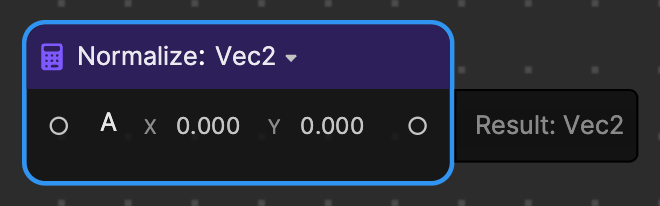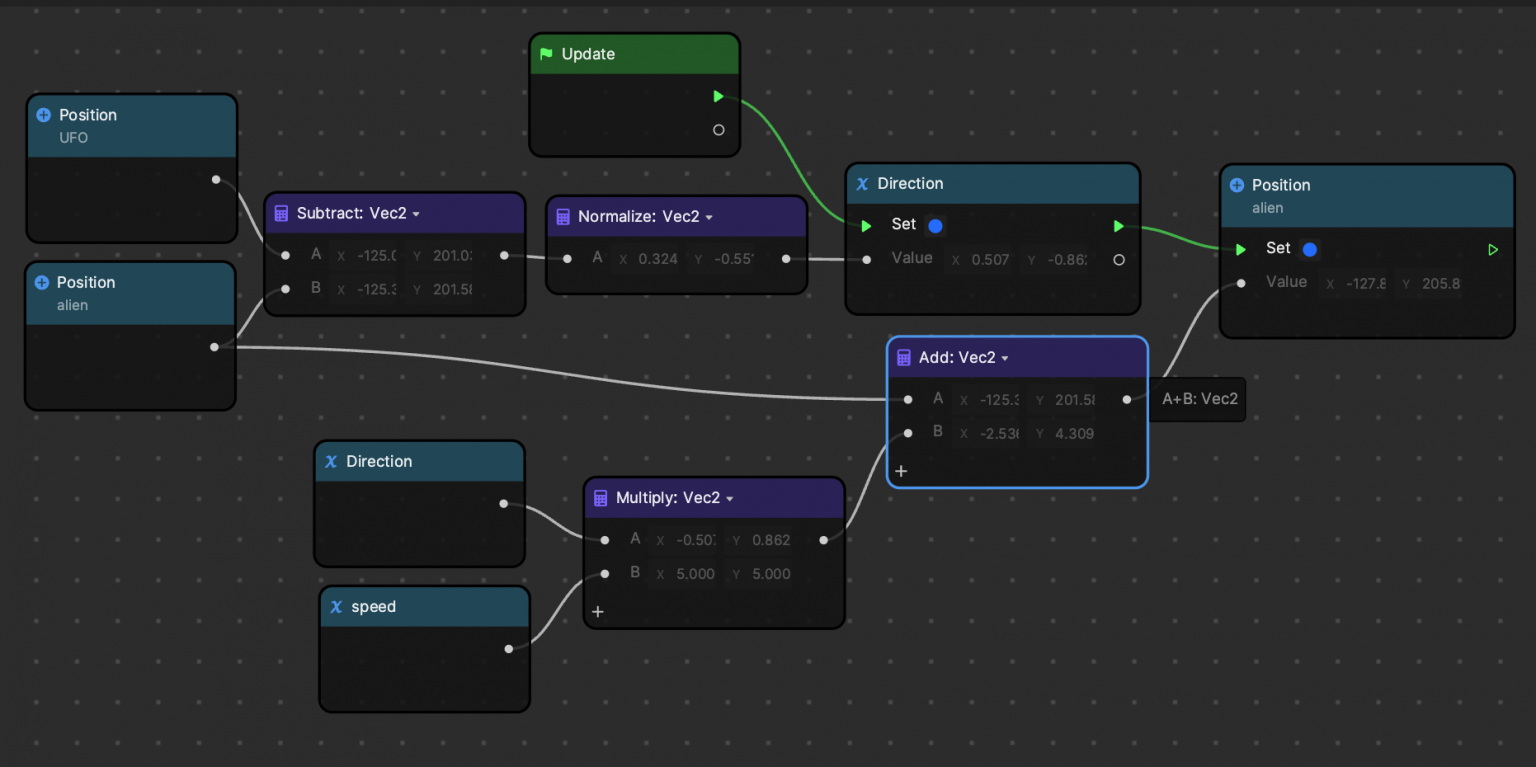Version: 3.3.0

# NormalizeUse Normalize to normalize the input vector. That is, return a vector of the same direction as the input with a length of 1. Supported types: Vec2, Vec3, and Vec4. Normalize is in the Math category.

## Input​

NameData TypeDescription
AVec4The vector to be normalized.

## Output​

NameData TypeDescription
ResultVec4The normalized vector with a length of 1.

## Example​This example calculates and gets the normalized direction from the alien to the UFO, then transforms the alien to move towards the UFO‘s direction at a uniform speed.